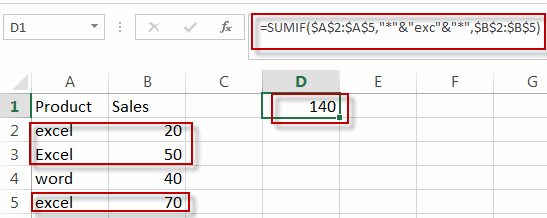# SUMIF When Partial Text String Match

This post will guide you how to sum the list of cells based on partial match using SUMIF function in excel. How to sumif cell values when partial text string matches in excel. How to sum cells based on partial dates, numbers, and text string that match specific criteria in excel.

## SUMIF When Partial Text String Match

If you want to sum cell values in column B and that match one criteria that the cell in the same row contains the partial text string “exc” in column A. You need to create a new formula based on the SUMIF function to achieve the result. Just do the following steps:

#1 type the following formula in the formula box of cell D1, then press enter key in your keyboard.

=SUMIF(\$A\$2:\$A\$5,”*”&”exc”&”*”,\$B\$2:\$B\$5)

#2 Let’s see the result.### Related Functions

• Excel SUMIF Function
The Excel SUMIF function sum the numbers in the range of cells that meet a single criteria that you specify. The syntax of the SUMIF function is as below:=SUMIF (range, criteria, [sum_range])…